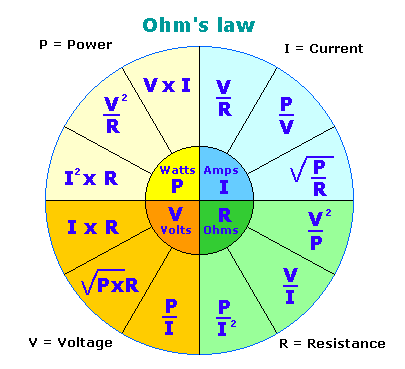# Ohm’s Law

If you are going to learn anything about electric circuit principles then Ohm’s Law should be top of your list. With Ohm’s Law you can make calculations to quantify the power, voltage, current, and resistance in renewable energy systems with ease.Above is a handy chart showing all of the inter-relationships beween power, current, voltage, and resistance in which:

V is measured in Volts.
R is measured in Ohms.
I is measured in Amps.
P is measured in Watts.

Below is an introductory video guide to Ohm’s Law which starts by explaining what Voltage, Current, and Resistance actually mean with clear analogies to aid understanding: